Definitions of Square Dance Calls and Concepts
Secondly | Thirdly | Fourthly {anyConcept} {anything} [C3B]

Index -->  Plus  |  A1  |  A2  |  C1  |  C2  |  C3A  |  C3B  |  C4  |  NOL  |
Definitions (Text Only) -->  Plus  |  A1  |  A2  |  C1  |  C2  |  C3A  |  C3B  |  C4  |  NOL  |
 Find call:

Secondly | Thirdly | Fourthly anyConcept anything -- [C3B]

 \$B8@8l(BBreak up the anything call into its component parts. Do the anything call normally until the given part; then do the given part applying the given CONCEPT then do the remaining parts of the anything call normally. EN: 10
\$B;X<(\$5\$l\$?(B anything \$B\$N%3!<%k\$r(B, \$B9=@.MWAG\$N%Q!<%H\$KJ,2r\$7\$^\$9(B. \$B;X<(\$5\$l\$?%Q!<%H\$NA0\$^\$G(B anything \$B\$N%3!<%k\$rIaDL\$K9T\$\$(B; \$B\$=\$7\$F(B, \$B;X<(\$5\$l\$?(B CONCEPT \$B\$G;X<(\$5\$l\$?%Q!<%H\$r9T\$\$(B, \$B\$=\$7\$F(B anything \$B\$N%3!<%k\$N;D\$j\$N%Q!<%H\$rIaDL\$K9T\$\$\$^\$9(B. JP: 10

\$BCm
• Only apply the given CONCEPT to the given part. I.e., EN: 20
\$B;X<(\$5\$l\$?(B CONCEPT \$B\$r;X<(\$5\$l\$?%Q!<%H\$@\$1\$K;H\$\$\$^\$9(B. \$B\$9\$J\$o\$A(B, JP: 20
• Secondly: only apply the concept to the second part. EN: 22
Secondly: \$B;X<(\$5\$l\$?%3%s%;%W%H\$r(B 2 \$BHVL\(B (second) \$B\$N%Q!<%H\$@\$1\$K;H\$\$\$^\$9(B. JP: 22
• Thirdly: only apply the concept to the third part. EN: 24
Thirdly: \$B;X<(\$5\$l\$?%3%s%;%W%H\$r(B 3 \$BHVL\(B (third) \$B\$N%Q!<%H\$@\$1\$K;H\$\$\$^\$9(B. JP: 24
• Fourthly: only apply the concept to the fourth part. EN: 26
Fourthly: \$B;X<(\$5\$l\$?%3%s%;%W%H\$r(B 4 \$BHVL\(B (fourth) \$B\$N%Q!<%H\$@\$1\$K;H\$\$\$^\$9(B. JP: 26
• Also possible, but rarely used: Fifthly, Sixthly, Seventhly (e.g., Seventhly Twisted Eight Chain Thru). There are very few calls that have more than 4 parts. EN: 30
\$B\$^\$?2DG=\$G\$9\$,\$[\$H\$s\$I;H\$o\$l\$J\$\$\$b\$N\$H\$7\$F(B: Fifthly, Sixthly, Seventhly (\$BNc\$(\$P(B, Seventhly Twisted Eight Chain Thru) \$B\$,\$"\$j\$^\$9(B. 5 \$B0J>e\$N%Q!<%H\$N%3!<%k\$O(B, \$B\$H\$F\$b>/\$J\$\$\$G\$9(B. JP: 30
• Do the call but when you reach the given part, re-evaluate and apply the given CONCEPT to the given part, then re-evaluate your position in the resulting formation and do the remainder of the call. EN: 40
\$B%3!<%k\$r9T\$\$(B, \$B;X<(\$5\$l\$?%Q!<%H\$H\$J\$C\$?\$i(B, re-evaluate (\$B:FI>2A(B, \$B8+D>\$9\$3\$H(B) \$B\$r\$7(B, \$B;X<(\$5\$l\$?(B CONCEPT \$B\$G;X<(\$5\$l\$?%Q!<%H\$r9T\$\$(B, \$B\$=\$7\$F(B, \$B7k2L\$N(B formation \$B\$G\$N<+J,\$N0LCV\$r(B re-evaluate \$B\$7\$F(B, \$B%3!<%k\$N;D\$j\$r9T\$\$\$^\$9(B. update JP: 40
• Secondly | Thirdly | Fourthly are Meta Concepts. A Meta Concept applies to another concept, not a call. The caller can not say Secondly anything where anything is just a call. The caller must give a concept after the word SecondlyEN: 50
Secondly | Thirdly | Fourthly \$B\$O(B Meta Concept \$B\$G\$9(B. Meta Concept \$B\$Nanything \$B\$,C1\$J\$k%3!<%k\$N\$H\$-(B, Secondly anything \$B\$H%3!<%k\$9\$k\$3\$H\$O\$G\$-\$^\$;\$s(B. \$B%3!<%i!<\$O(B, Secondly \$B\$N8e\$K(B concept \$B\$r;X<(\$7\$J\$1\$l\$P\$J\$j\$^\$;\$s(B. JP: 50
• Sometimes, the given concept may not "feel" like a concept. E.g., Secondly Boys, or Thirdly Twice. For the purposes of Secondly|Thirdly|Fourthly, anything that modifies the call is considered a concept. This includes identifiers (e.g., Boys, Girls, Ends, Centers, Leaders, Trailers, Beaus, Belles, Heads, Sides); formations (e.g., Triple Box); numerics (e.g., twice, 1 & 1/2, 2/3); and other modifiers (e.g., Transfer And, Tally Ho But). EN: 60
\$B;X<(\$5\$l\$?(B concept \$B\$,(B concept \$B\$G\$J\$\$\$h\$&\$K46\$8\$k\$3\$H\$b\$"\$j\$^\$9(B. \$BNc\$(\$P(B, Secondly Boys \$B\$d(B Thirdly Twice \$B\$G\$9(B. Secondly|Thirdly|Fourthly \$B\$NL\E*\$K\$h\$j(B, \$B%3!<%k\$r=\$>~\$9\$k\$b\$N\$OA4\$F(B concept \$B\$H9M\$(\$^\$9(B. \$B\$3\$l\$i\$K\$O(B, \$B?M\$N;X<((B (\$BNc\$(\$P(B, Boys, Girls, Ends, Centers, Leaders, Trailers, Beaus, Belles, Heads, Sides); formations (\$BNc\$(\$P(B, Triple Box); \$B?t(B (\$BNc\$(\$P(B, twice, 1 & 1/2, 2/3); \$B\$=\$NB>(B (\$BNc\$(\$P(B, Transfer And, Tally Ho But) \$B\$J\$I\$,\$"\$j\$^\$9(B. JP: 60
• It is important to re-evaluate your position both before and immediately after the given part as, depending upon the given concept, you may unexpectedly be working with different dancers or in a different formation. EN: 70
\$B;X<(\$5\$l\$?%Q!<%H\$NA0\$HD>8e\$G(B, \$B;X<(\$5\$l\$?(B concept \$B\$K\$h\$j(B, \$B<+J,\$N0LCV\$r(B re-evaluate (\$B:FI>2A(B, \$B8+D>\$9\$3\$H(B) \$B\$9\$k\$3\$H\$,Bg@Z\$G\$9(B. \$B9M\$(\$b\$D\$+\$J\$\$?M\$d(B formation \$B\$GF0\$/\$3\$H\$K\$J\$k\$3\$H\$,\$"\$j\$^\$9(B. update JP: 70
• Secondly Tandem Reset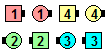Secondly Tandem Reset\$B\$NA0(B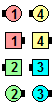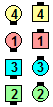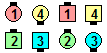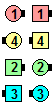1/2 Zoom\$B\$N8e(B(1/4) Tandem Hinge\$B\$N8e(B(1/2) 1/2 Zoom\$B\$N8e(B(3/4) Hinge\$B\$N8e(B (\$B=*\$o\$j(B)
Thirdly Tandem Reset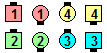Thirdly Tandem Reset\$B\$NA0(B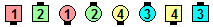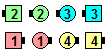1/2 Zoom\$B\$N8e(B(1/4) Hinge\$B\$N8e(B(1/2)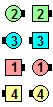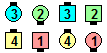Tandem 1/2 Zoom\$B\$N8e(B(3/4) Hinge\$B\$N8e(B (\$B=*\$o\$j(B)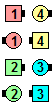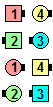Fourthly Tandem Swing The Fractions\$B\$NA0(B Right Arm Turn 1/4\$B\$N8e(B(1/5) Left Arm Turn 1/2\$B\$N8e(B(2/5)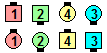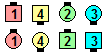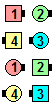Right Arm Turn 3/4\$B\$N8e(B(3/5) Tandem Left Arm Turn 1/2\$B\$N8e(B(4/5) Right Arm Turn 1/4\$B\$N8e(B (\$B=*\$o\$j(B)

Initially Concept [C3A] (Vic Ceder 1994):
Do the first part of the anything call applying the given CONCEPT; then do the remainder of the anything call without the given CONCEPTEN: 327
\$B;X<(\$5\$l\$?(B anything \$B\$N%3!<%k\$N;O\$a\$N%Q!<%H\$r(B, \$B;X<(\$5\$l\$?(B CONCEPT \$B\$G9T\$\$(B; anything \$B\$N%3!<%k\$N;D\$j\$O(B, \$B;X<(\$5\$l\$?(B CONCEPT \$B\$r;H\$o\$:\$K9T\$\$\$^\$9(B. JP: 327

Finally Concept [C3A] (Vic Ceder 1994):
Do the entire anything call applying the given CONCEPT only to the last part of the anything call. EN: 319
\$B;X<(\$5\$l\$?(B anything \$B\$N%3!<%k\$NA4BN\$r9T\$\$\$^\$9\$,(B; \$B;X<(\$5\$l\$?(B CONCEPT \$B\$O(B, \$B;X<(\$5\$l\$?(B anything \$B\$N%3!<%k\$N:G8e\$N%Q!<%H\$@\$1\$K;H\$\$\$^\$9(B. JP: 319

Oddly Concept [C3B] :
Do the odd-numbered parts applying any CONCEPT and the even-numbered parts normally. EN: 837
\$B;X<(\$5\$l\$?%3!<%k\$N4q?t%Q!<%H\$O(B, \$B;X<(\$5\$l\$?(B CONCEPT \$B\$G9T\$\$(B; \$B6v?t\$N%Q!<%H\$OIaDL\$K9T\$\$\$^\$9(B. JP: 837

Evenly CONCEPT [C3B] :
Do the even-numbered parts applying any CONCEPT and the odd-numbered parts normally. EN: 838
\$B;X<(\$5\$l\$?%3!<%k\$N6v?t%Q!<%H\$O(B, \$B;X<(\$5\$l\$?(B CONCEPT \$B\$G9T\$\$(B; \$B4q?t\$N%Q!<%H\$OIaDL\$K9T\$\$\$^\$9(B. JP: 838

Initially | Secondly | etc Use A(n) anything1 For A(n) anything2 [C4]:
Replace the given part of the anything2 call with the anything1 call. E.g., from R-H Columns: Thirdly Use An Ah So for a Swing The Fractions: all Right Arm Turn 1/4; those who can left Arm Turn 1/2; everybody Ah So; those who can Left Arm Turn 1/2; all Right Arm Turn 1/4. EN: 839
\$B;X<(\$5\$l\$?(B anything2 \$B\$N%3!<%k\$N;X<(\$5\$l\$?%Q!<%H\$r(B, \$B;X<(\$5\$l\$?(B anything1 \$B\$N%3!<%k\$G(B replace \$B\$7\$^\$9(B. \$BNc\$(\$P(B, \$B1&Thirdly Use An Ah So for a Swing The Fractions \$B\$O(B: all Right Arm Turn 1/4; those who can left Arm Turn 1/2; everybody Ah So; those who can Left Arm Turn 1/2; all Right Arm Turn 1/4 \$B\$G\$9(B. JP: 839

\$BAppendix C: Calls with PartsChoreography for Secondly | Thirdly | Fourthly anyConcept anythingComments? Questions? Suggestions?

https://www.ceder.net/def/secondly.php?level=master&language=japan&action=edit
29-January-2020 11:24:56